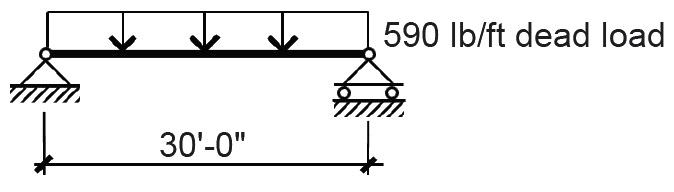Jonathan Ochshorn's Structural Elements for Architects and Builders, Third Edition
contact | structural element calculators | paperback and pdf | « previous section | next section » | contents

Dead loads consist of the weight of the building itself, including structure, partitions, cladding, roofing materials and permanent interior finishes such as carpet, ceiling systems, etc. These gravity loads are always downward-acting, and can be calculated with a reasonable degree of accuracy, being the summation of various building material weights, which are easily determined and quite predictable. That being said, it is sometimes prudent to anticipate unpredictable scenarios which call for additional dead load, so that future building modifications (such as the addition of a heavy tile floor, or a change from a mechanically attached to a ballasted roof) can be made without major structural modifications.

Dead loads are calculated by multiplying the unit weight of the materials by their quantity. Weights of some common materials and assemblies are listed in Appendix Table A-2.1.

Calculator

Problem definition. Assume a typical steel structure with corrugated steel deck and concrete slab, tile floor, suspended ceiling system, and allowances for partitions and mechanical ducts, as shown in Figure 2.1. The spandrel girders carry an additional cladding load consisting of a brick and block cavity wall, 12-ft high from floor to floor. Find the dead load distribution on beam A and spandrel girder B.Figure 2.1: Framing plan for Example 2.1

Solution overview. Find weights of building elements; compute total dead load on beams and girders.

Problem solution

Beam A

1. From Appendix Table A-2.1, find weights of building elements:

a. steel deck, finish floor, ducts and ceiling system = 47 psf.

b. partitions = 8 psf.

c. subtotal = 55 psf.

2. Compute weight per linear foot of beam by multiplying unit weight by tributary area on one linear foot of the beam: 55 × 10 = 550 lb/ft.

3. From Appendix Table A-2.1, assume weight of beam: 40 lb/ft.Figure 2.2: Beam A load diagram for Example 2.1

Girder B

1. Find concentrated dead loads at third points caused by typical beam reactions, equal to the distributed load on the beam times the beam span divided by two: P = 590(30)/2 = 8850 lb.

2. From Appendix Table A-2.1, find weight of cladding = 1000 lb/ft.

3. From Appendix Table A-2.1, assume weight of girder: 80 lb/ft.

4. Add girder weight to cladding weight = 80 + 1000 = 1080 lb/ft.